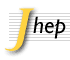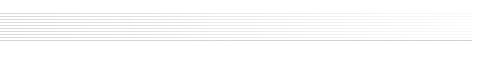General Manuscript preparation General information Text and style Tables and figures Supplementary material attached Manuals and computer codes References File preparation TeX, LaTeX LaTeX tips Archive preparation (tar.gz) Submission F.A.Q. After Submission Coauthors Select Coauthor Switch Corresponding Author The preprint web page Proof-reading and publication Copyright statement Access to your paper

## Keyword List

 Note The keywords are organized in sections. The section name is highlighted in grey followed by the list of individual keywords belonging to that section.

 Group: Experimental
Collider Physics Underground and Large Array Physics
• e+-e- Experiments
• Heavy Ion Experiments
• Lepton-Nucleon Scattering (experiments)
• Dark Matter and Double Beta Decay (experiments)
• Gamma and Cosmic Rays (experiments)
• Neutrino Detectors and Telescopes (experiments)
Non collider experiments with beams Optional keywords (supplementary)
• Fixed target experiments
• Other experiments
• Jet substructure
• B physics
• Beyond Standard Model
• Branching fraction
• Charm physics
• chiral b'
• CKM angle alpha
• CKM angle beta
• CKM angle gamma
• Collective flow
• CP violation
• Dark matter
• Diffraction
• Electroweak interaction
• Event-by-event fluctuation
• Exotics
• FCNC Interaction
• Flavor physics
• Flavour Changing Neutral Currents
• Forward physics
• four-top-production
• Global features
• Hard scattering
• harmonic flow
• Heavy quark production
• Heavy-ion collision
• Higgs physics
• Jet physics
• Jets
• Lepton production
• Minimum bias
• Oscillation
• Particle and resonance production
• Particle correlations and fluctuations
• Phase transitions
• Photon production
• Polarization
• proton-proton scattering
• QCD
• Quark deconfinement
• Quark gluon plasma
• Quarkonium
• Rare decay
• Relativistic heavy ion physics
• same charge top pair
• Spectroscopy
• Supersymmetry
• Tau Physics
• Top physics
• top squark
• Unfolding
• vector-like quarks
 Group: Theoretical
Quantum Field Theory Gauge Field Theories
• BRST Quantization
• Conformal Field Theory
• Effective Field Theories
• Field Theories in Higher Dimensions
• Field Theories in Lower Dimensions
• Large Extra Dimensions
• Lattice Quantum Field Theory
• Nonperturbative Effects
• Renormalization Group
• Renormalization Regularization and Renormalons
• Sigma Models
• Solitons Monopoles and Instantons
• Supersymmetric Gauge Theory
• Topological Field Theories
• 1/N Expansion
• Anyons
• Chern-Simons Theories
• Confinement
• Duality in Gauge Field Theories
• Scattering Amplitudes
• Spontaneous Symmetry Breaking
• Topological States of Matter
• Wilson, 't Hooft and Polyakov loops
Symmetries String and Brane Theory
• Anomalies in Field and String Theories
• Conformal and W Symmetry
• Discrete Symmetries
• Gauge Symmetry
• Global Symmetries
• Higher Spin Symmetry
• Space-Time Symmetries
• Black Holes in String Theory
• Bosonic Strings
• Brane Dynamics in Gauge Theories
• Conformal Field Models in String Theory
• D-branes
• F-Theory
• Flux compactifications
• Gauge-gravity correspondence
• Holography and condensed matter physics (AdS/CMT)
• Holography and quark-gluon plasmas
• Long strings
• M-Theory
• M(atrix) Theories
• p-branes
• String Duality
• String Field Theory
• String theory and cosmic strings
• Superstring Vacua
• Superstrings and Heterotic Strings
• Tachyon Condensation
• Topological Strings
General Relativity and Gravitation Supersymmetry
• 2D Gravity
• Black Holes
• Classical Theories of Gravity
• Higher Spin Gravity
• Lattice Models of Gravity
• Models of Quantum Gravity
• Spacetime Singularities
• Extended Supersymmetry
• Supergravity Models
• Superspaces
• Supersymmetric Effective Theories
• Supersymmetry and Duality
• Supersymmetry Breaking
Mathematical Methods of Physics Mostly Solvable Models
• Differential and Algebraic Geometry
• Integrable Hierarchies
• Non-Commutative Geometry
• Quantum Groups
• Stochastic Processes
• Integrable Field Theories
• Lattice Integrable Models
• Matrix Models
Astroparticles Statistical Field Theories
• Cosmology of Theories beyond the SM
• Solar and Atmospheric Neutrinos
• Thermal Field Theory
• Bethe Ansatz
• Boundary Quantum Field Theory
• Quantum Dissipative Systems
• Random Systems
Mostly Weak Interactions Mostly Strong Interactions
• Beyond Standard Model
• Compactification and String Models
• CP violation
• GUT
• Heavy Quark Physics
• Higgs Physics
• Kaon Physics
• Neutrino Physics
• Precision QED
• Quark Masses and SM Parameters
• Supersymmetric Standard Model
• Technicolor and Composite Models
• Chiral Lagrangians
• Lattice QCD
• Perturbative QCD
• Phase Diagram of QCD
• Quark-Gluon Plasma
• Resummation
 Group: Phenomenological
Quantum Field Theory (phenomenology) Strings and branes
• Lattice field theory simulation
• Non-perturbative renormalization
• Phenomenology of Field Theories in Higher Dimensions
• Phenomenology of Large extra dimensions
• Strings and branes phenomenology
Phenomenological Aspects of Supersymmetry Mostly Strong Interactions (phenomenology)
• Supersymmetry Phenomenology
• Deep Inelastic Scattering (Phenomenology)
• Heavy Ion Phenomenology
• Jets
• NLO Computations
• Phenomenological Models
• QCD Phenomenology